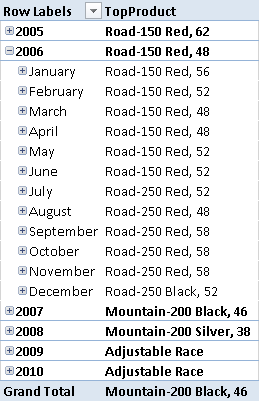The FIRSTNONBLANK LASTNONBLANK functions return the first and last item, respectively, of the table passed as first argument that returns a non-blank result for the expression passed as second argument. The syntax reported in the help on line is:

```FIRSTNONBLANK ( <column>, <expression> )
LASTNONBLANK ( <column>, <expression> )
```

Thus, you usually write a formula that use the date column of a Date table as first argument, as in the following example.

```Units LastNonBlank :=
CALCULATE (
SUM ( Inventory[UnitsBalance] ),
LASTNONBLANK (
'Date'[Date],
CALCULATE ( SUM ( Inventory[UnitsBalance] ) )
)
)
```

However, the correct definition of the two functions is the one where the first argument specifies a table having only one column.

```FIRSTNONBLANK ( <table_single_column>, <expression> )
LASTNONBLANK ( <table_single_column>, <expression> )
```

In practice, specifying a column name in the first argument is just syntax sugar, because instead of:

```LASTNONBLANK ( 'Date'[Date], <expression> )
```

you can write the equivalent:

```LASTNONBLANK ( VALUES ( 'Date'[Date] ), <expression> )
```

You can also use a different table function, such as ALL or FILTER. Why is this important? Because in DAX usually you cannot make assumptions on sort order of values in a table or in a column, unless you use specific functions. FIRSTNONBLANK and LASTNONBLANK are two DAX functions that iterate the values of a column based on their native sort order (so the data type of the column is relevant on this regard). Moreover, you can use FIRSTNONBLANK and LASTNONBLANK with any data type, not just date.

One scenario of this usage is when you need to retrieve a single value for a measure. You can use MIN and MAX only with numeric data types. Therefore, you can use FIRSTNONBLANK instead of MIN, and LASTNONBLANK instead of MAX – manipulating also text columns in this way. Simply put a constant expression in the second argument in this case.

```MIN ( <column> ) = FIRSTNONBLANK ( <column>, 1 )
MAX ( <column> ) = LASTNONBLANK ( <column>, 1 )
```

Another scenario is using TOPN in a measure, returning the first product or customer based on sales. For example, consider the following measure:

```TopProduct :=
TOPN (
1,
VALUES ( Product[Product Name] ),
[Internet Total Sales]
)
```

The TopProduct measure will generate an error in case there is a tie between two or more products with the same top value of sales amount. In this case, TOPN returns a table with one column and two or more rows, producing an error in the measure. You can fix this by getting the first product between those in tie, according to alphabetical order.

```TopProduct :=
FIRSTNONBLANK (
TOPN (
1,
VALUES ( Product[Product Name] ),
[Internet Total Sales]
),
1
)
```

You can see an example of the TopProduct measure applied to year and months in the following picture.There are certainly other scenarios where using FIRSTNONBLANK and LASTNONBLANK could be useful. It is important to remember that these functions have a special behavior in DAX, using the sort order of the values in a column, regardless of its data type.